×#### Thank you for registering.

One of our academic counsellors will contact you within 1 working day.

Click to Chat

1800-1023-196

+91-120-4616500

CART 0

• 0

MY CART (5)

Use Coupon: CART20 and get 20% off on all online Study Material

ITEM
DETAILS
MRP
DISCOUNT
FINAL PRICE
Total Price: Rs.

There are no items in this cart.
Continue Shopping```Rate of Change of Quantities

Table of Content

Introduction to Rate of Change of Quantities

What is Rate of Change?

Rate of Change of quantity

Two variables varies with respect to another variable

Positive or Negative Rate of Change

Related Rate

Examples

Introduction to Rate of Change of Quantities

Rate of change of quantity is one of the most important application of derivatives as the concept of derivative it comes from the rate of change.

This is used in our daily activities as the change is all over around us like change in the price of quantities, change in the number of population etc.

In geometry, it is called the Slope, which tells us the rate of change in the slope of curve that is, the change in y with respect to x.

What is Rate of Change?

Rate of change is a rate which tells us that how one quantity changes with respect to another quantity.Here in the above figure, it is shown that how much height of the baby is increasing with the increase in time.

Here time is the independent variable and baby’s height is the dependent variable as the increase in height is dependent on the change in time.

If x is an independent variable and y is a dependent variable thenThis is the ratio which tells us the change in y with respect to change in x. this is called the Average Change.

Rate of Change of quantity

As we already know the formula of velocity that is,This formula is actually the derivative which tells us the respective change in distance with respect to time i.e. how much distance is covered as the time increases.

Similarly, if we have a function say y = f(x) and one quantity y varies with another quantity x then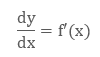shows the rate of change of y with respect to x and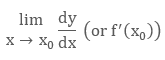represents the rate of change of y with respect to x at x = x0.

This is called the Instantaneous Rate of Change as we are calculating derivatives at a particular interval. In the application of derivatives we generally use the instantaneous rate of change.

Two variables varies with respect to another variable

If there are two variables likes x and y are varying with respect to another variable‘s’ so that

x = f(s) and y = g(s), then we will use the chain rule to solve it.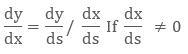Hence, the rate of change of y in relation with x can be calculated using the rate of change of y and that of x both with respect to t.

Positive or Negative Rate of Change

dy/dx Could be positive or negative. It is related with the increase or decrease in the y variable with respect to other variable. And if a quantity does not change, it is called Zero Rate of Change.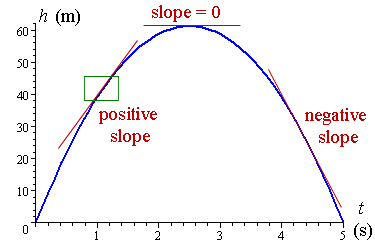Positive Rate of Change: When the value of y increases with the increase in the value of x, then dy/dx will be positive that is, the graph of the function moves upwards.

Negative Rate of Change: When the value of y decreases with the increase in the value of x, then dy/dx will be negative that is, the graph of the function moves downwards.

Zero Rate of Change: When the value of y remains constant with the increase or decrease of x, then dy/dx will be 0 that is, the graph of the function will remain same.

Example:

For what values of x are the rate of increase of function y = x3 - 5x2 + 5x + 8 is twice the rate of increase of x?

Solution:

Given the equation

y = x3- 5x2 + 5x + 8

Rate of increase is given that function y is increasing twice the increase of x.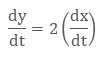Differentiate both sides with respect to time "t",Put the given information of rate of change in the equation 1Hence, the values of x are 1/3 or 3 at which the rate of increase of y is twice the rate of increase of x.

Related Rate

Related Rate is the rate which tells the relation of one variable with respect to time and it helps us to solve the problems easily.

If we know the rate of change then we can easily find the relation between two variables.

Examples

Example 1:

If a circular sheet of metal is heated then its radius will increase at the rate of 1/4 cm/sec. At what rate, the sheet’s area will increase when the radius is 40 cm?

Solution: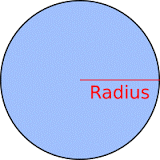Given

Radius = 40 cm (r = 40)

Rate at which radius is increasing = dr/dt = 1/4

Area of circle = A = πr2Substitute the values in the derivative of area of circle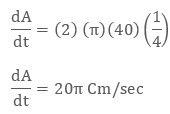Example 2:

We have a rectangular field whose length is changing at the rate of 4 m/s and the perimeter is changing at a rate of 12 m/s at an instant of time. At what rate is the width changing at that instant? At what rate is the area changing at that instant?

Solution:Given

Rate of change of length = dl/dt =4

Rate of change of perimeter = dP/dt =12

We know the formula of the Perimeter of rectangular field:

P = 2l + 2w

Now we will differentiate the formula of perimeter: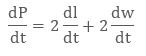Substitute the given information in the differentiated equation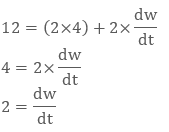Hence, the width is changing at a rate of 2 m/s.

Example 3:

A particle moves along the curve 6y = x3 + 2, find the points on the curve at which y coordinate is changing 8 times as fast as x coordinate.

Solution:

GivenThis shows that the change in y coordinate is 8 times as fast as x coordinate.

6y = x3 + 2 ……….(2)

Now we will differentiate the equation of the curve with respect to time.Now we have to find the points on the curve which fulfills the above condition.

When x = 4

6y = x3 + 2

6y = 43 + 2

6y = 64 + 2

y = 66/6

y = 11

When x = – 4

6y = x3 + 2

6y = (-4)3 + 2

6y = – 64 + 2

y = – 62/6

y = -31/3

Hence the two points on the curve where the y coordinate is increasing 8 times faster than the x coordinates are (4, 11) and (-4,- 31/3)

Example 4:

A man 2m high walks, at a uniform speed of 6m/min away from a lamp post, 5m high. Find the rate at which the length of the man’s shadow increasing.

Solution:Given

Height of man = EC = 2m

Height of lamp post = AB = 5m

CD = y

BD = x+y

Rate of change in x that is, the rate of change in speed of man with respect to time is given 6 mt/min.Here two similar triangles ∆ABD ≈ ∆ECD are given.

According to the property of similar triangles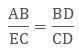Now substitute the given values in the above fraction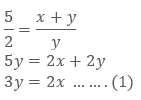Now we will differentiate the equation 1 with respect to time as the shadow is increasing with respect to time.Now we will directly put the value of dx/dt in the above differentiation.Hence the rate of change in shadow of man is 4 m/min.

Watch this Video for more reference

Rate of Change of Quantities
```### Course Features

• 731 Video Lectures
• Revision Notes
• Previous Year Papers
• Mind Map
• Study Planner
• NCERT Solutions
• Discussion Forum
• Test paper with Video Solution